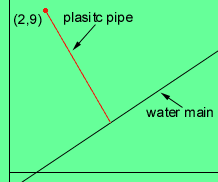From: Vanja Hello, My question is...A house is to be connected to a new water main that runs along the line y=2/3x-1. The connection point at the house has coordinates (2,9), where the units represent metres. What lenght of plastic pipe is needed to connect to the water main at the closest point? Hi Vanja, I would first draw a diagram. Draw the coordinate plane, the line y=2/3x-1, and the point (2,9). If the plastic pipe is to meet the water line at the closest point then they meet each other in a right angle. The slope of the line y=2/3x-1 is 2/3 and thus the slope of the slope of the line representing the plastic pipe must be -3/2 if the two lines are perpendicular.Write the equation of the line through (2,9) with slope -3/2 and then find where this line intersects the line y=2/3x-1. Now the distance you want is the distance between this point of intersection and the point (2,9). Cheers, Penny Go to Math Central